Ex 5.3

Chapter 5 Class 10 Arithmetic Progressions
Serial order wise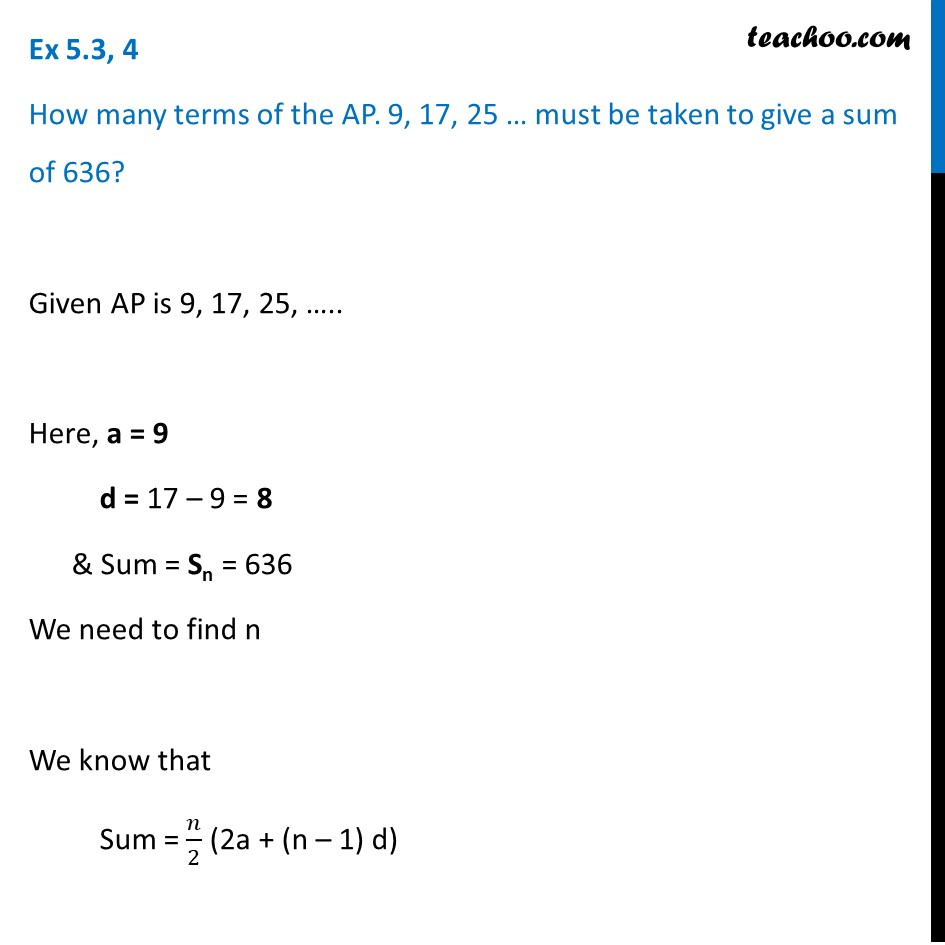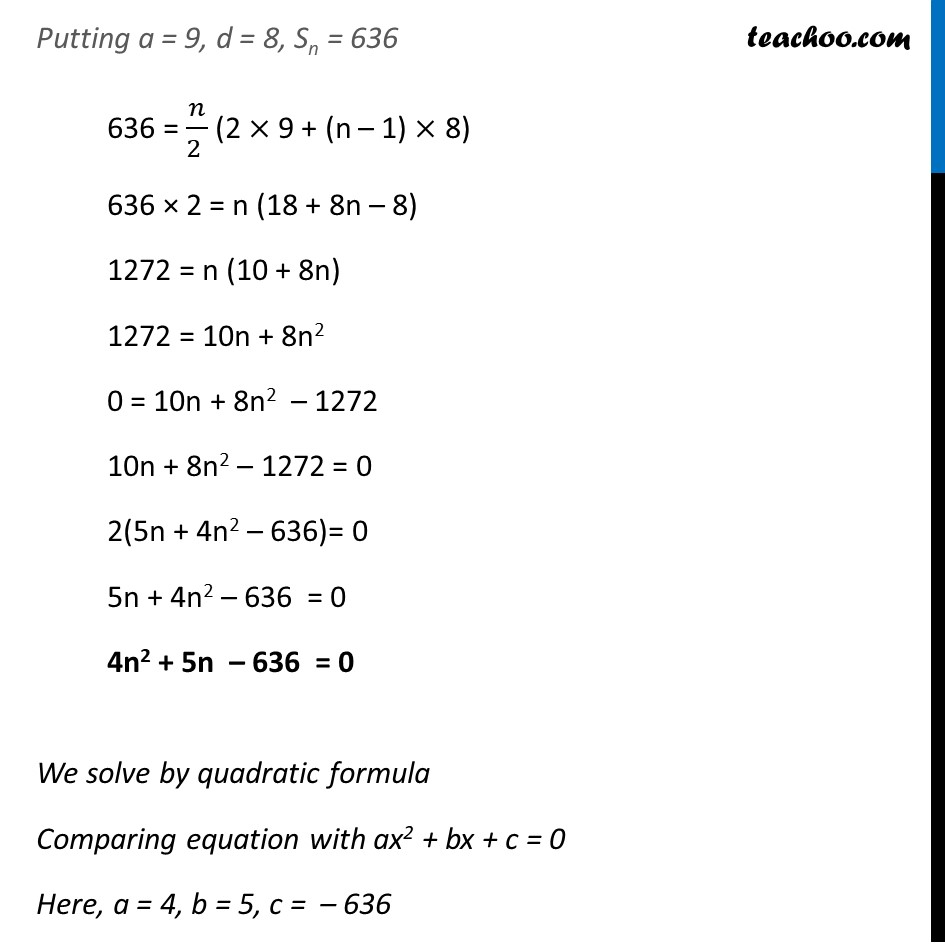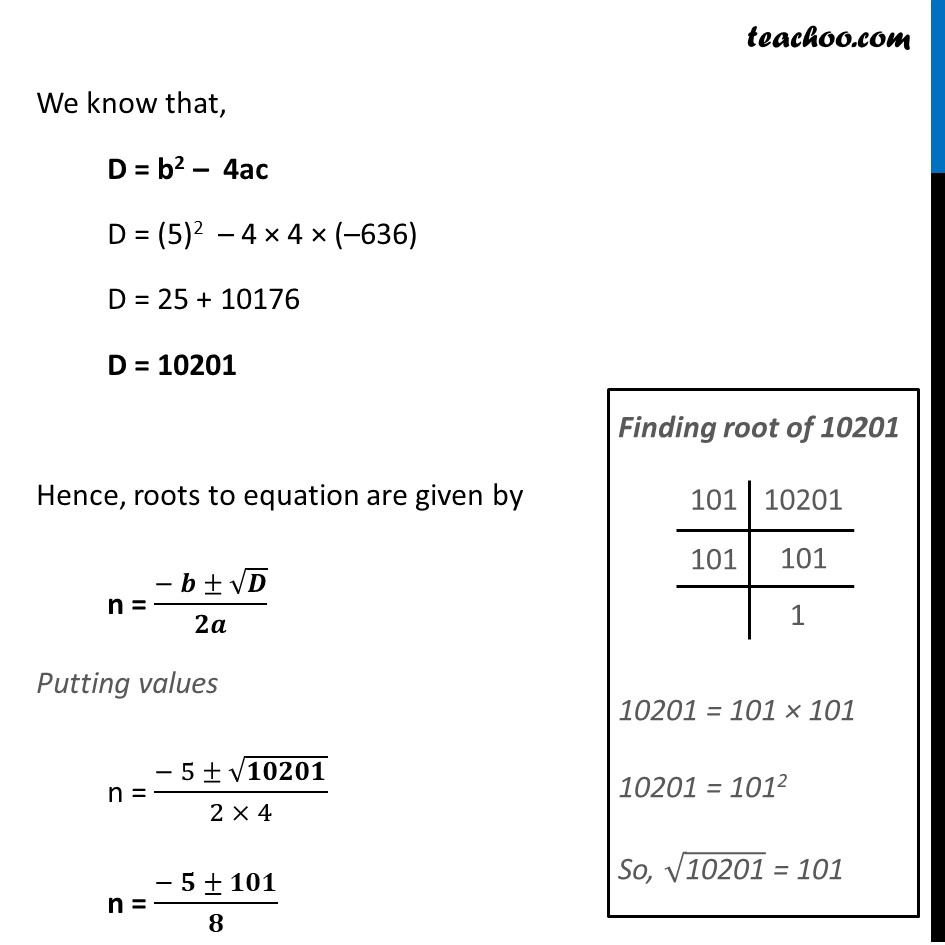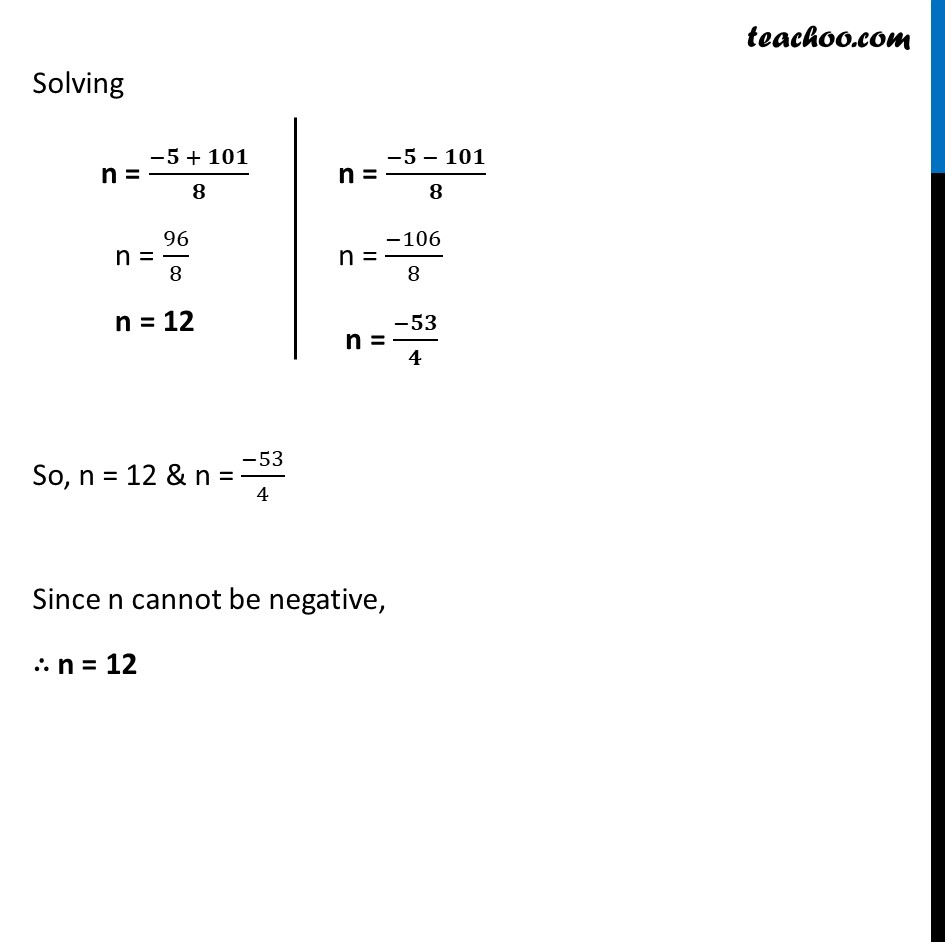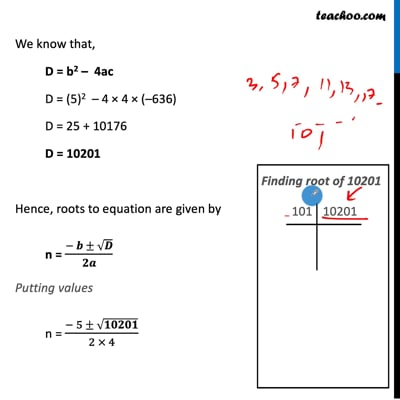This video is only available for Teachoo black users

Maths Crash Course - Live lectures + all videos + Real time Doubt solving!

### Transcript

Ex 5.3, 4 How many terms of the AP. 9, 17, 25 … must be taken to give a sum of 636? Given AP is 9, 17, 25, ….. Here, a = 9 d = 17 – 9 = 8 & Sum = Sn = 636 We need to find n We know that Sum = 𝑛/2 (2a + (n – 1) d) Putting a = 9, d = 8, Sn = 636 636 = 𝑛/(2 ) (2 × 9 + (n – 1) × 8) 636 × 2 = n (18 + 8n – 8) 1272 = n (10 + 8n) 1272 = 10n + 8n2 0 = 10n + 8n2 – 1272 10n + 8n2 – 1272 = 0 2(5n + 4n2 – 636)= 0 5n + 4n2 – 636 = 0 4n2 + 5n – 636 = 0 We solve by quadratic formula Comparing equation with ax2 + bx + c = 0 Here, a = 4, b = 5, c = – 636 We know that, D = b2 – 4ac D = (5)2 – 4 × 4 × (–636) D = 25 + 10176 D = 10201 Hence, roots to equation are given by n = (− 𝒃 ± √𝑫)/𝟐𝒂 Putting values n = (− 5 ± √𝟏𝟎𝟐𝟎𝟏)/(2 × 4) n = (− 𝟓 ± 𝟏𝟎𝟏)/𝟖 Finding root of 10201 10201 = 101 × 101 10201 = 1012 So, √("10201" ) = 101 Solving So, n = 12 & n = (−53)/4 Since n cannot be negative, ∴ n = 12 n = (−𝟓 + 𝟏𝟎𝟏)/𝟖 n = 96/8 n = 12 n = (−𝟓 − 𝟏𝟎𝟏)/𝟖 n = (−106)/8 n = (−𝟓𝟑)/𝟒